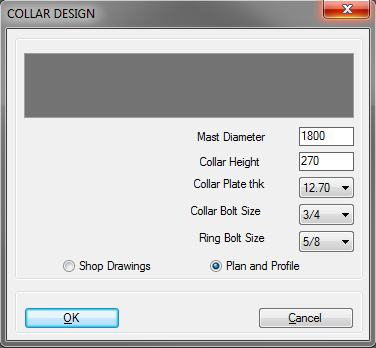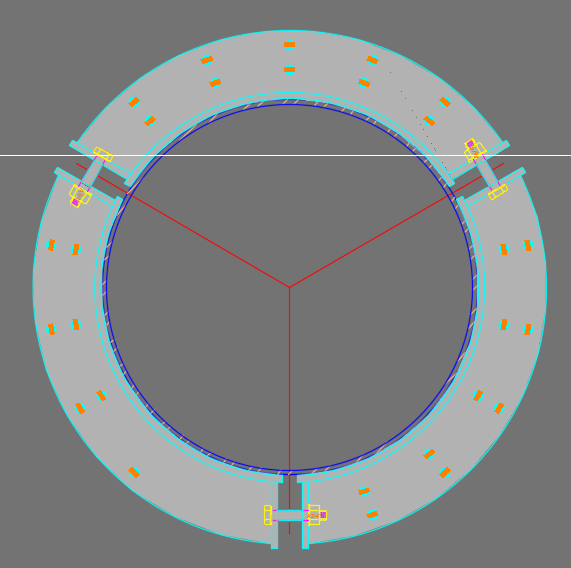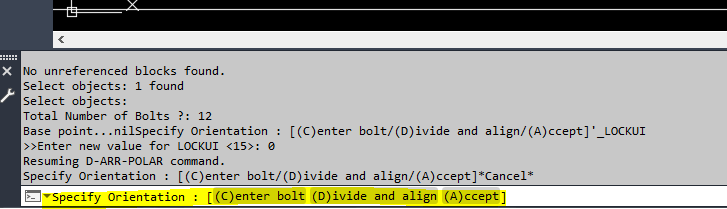# Steel Collar Design - Monopole

## Recommended PostsCollar Drawing.dwgHey Guys yet with another question:

I created this DCL that has 5 user input and I would like it to draw this complicated collar for me. Basically, the size of the mast (monopole diameter) is given together with the thickness of the collar plate (which is the same thickness for the ring plate), then there are some fixed values that can be assumed to draw this. Hopefully 1/3 can be drawn and then array at 120 deg. DWG I posted would be a better representative. The number of bolts (5 rows shown on this picture) is only the best fit for the support - I would like it to give me all options to choose from (3 bolt pattern all the way to 9 rows).

Then to make it even harder, I would like to create a shop drawing (as shown on dwg) that has detailed plan so it really should be drawn in 4 pieces (for that 1/3 of the pie) to then assign layer and hatch.

Can someone drag me to the right direction and show me how to draw stuff with different arcs and trims and offsets PLEASE!

I was so excited to finally show drop down menu on a DCL, now that is done, I do not know how to draw an arc with specific geometry in lisp.

Thanks

##### Share on other sitesIts a case of just coding every step you would do manually maybe turn "logfile on" then can see the command entries. Open in notepad and write code for each line.

A quick look draw a circle then offset circle 3 times. Remember each ring ie (setq r1 (entlast)) you may need this for trims.

Draw  1st 120 lines offset do trim r1 L & R

and so on.

For some use say (vla-offset obj offdist)  where offdist can be -ve for left so do twice for a left and right. 12 -12

```Circle
Select center point

(setq obj (vlax-ename->vla-object  (entlast)))
(vla-offset obj 10)
(vla-offset obj 18)
(vla-offset obj 42)
(vla-offset obj 50)```

Just start when stuck ask for help

##### Share on other sitesI would rather just create a dynamic block with all the array, stretch and scale properties and do the LSP code in one single line: vla-InsertBlock. Then using Lee's dynamic block functions, just set all the properties depending on DCL input.

##### Share on other sitesGood idea Jonathan, what dynamic blocks were really built for. Just do a 1/3rd then last is an array.

##### Share on other sitesIt is not a good idea in my case that the size of the circle is a dependant and the size of the ring bolt that determines the plate size specifies the size of the plate. It is a bit complicated than a simple stretch/move functions dynamic block offers. Also I cannot use parametric dimensions in Autocad since although I am creating it in Autocad, I want BricsCad users to use it too (BricsCad does not support block editing nor +90% of the dynamic block functionalities related to parametric restrains). I will try to pursue with writing my code that takes into account all the variations for this task.

Thanks

##### Share on other sitesSo far I was able to create the collar, ring and rung plate as I wanted. However I have a bit of an issue with:

1) making everything into a block (entities that are created by this routine).

2) I would like to avoid using macro commands if possible but have not yet get around xref group > entity names blah blah dissection yet. so I lazied up and used macro commands when I was stuck.

3) I would like to array certain bolt blocks depending on the size of bolt chosen, as shown on the photo I posted earlier, that should have two criteria: Degree between each bolt and number of bolts. I would like the array happens after the collar block is created.

any idea that can help me go through this part (meaningful help is appreciated)?

Please let me know if you can see a way to help me with those.

I will be using my DCL routine once everything is dandy but for now I am using getword function.

##### Share on other sites1 Old fashioned is probably easiest BLOCK pick all objects  base point etc keeping a list of every entity name could be done but easier just do last. As your creating it you should know the window that the part is in based on centre point and radius entries.

2 ?

3 Array polar for the bolts.

4 For any blocks like bolts use 0,0 as base point much easier to work out insertion point than some offset point.

##### Share on other sites33 minutes ago, BIGAL said:

1 Old fashioned is probably easiest BLOCK pick all objects  base point etc keeping a list of every entity name could be done but easier just do last. As your creating it you should know the window that the part is in based on centre point and radius entries.

2 ?

3 Array polar for the bolts.

4 For any blocks like bolts use 0,0 as base point much easier to work out insertion point than some offset point.

Hey @BIGAL,

‘could you write a short line for me as an example for a polar array using Vla polar please?

##### Share on other sitesJust google VLA-ARRAYPOLAR hint Afralisp. Even just use command your not doing thousands.

Edited by BIGAL

##### Share on other sitesCould someone help me with this please?

I am trying to create a getstring instead of pressing "V" then "enter an angle" to make the angle go 0.00 (center bolt) or 15.00 (divide) for example.

so each time I click in each string would toggle between 0 and 15 degrees and display as such.```;| ;;
DYNARRAY FUNCTION ;;
By: andrea Andreetti 2009-01-03 ;;
;;
Update 1.1 By A.A. 2009-01-03 ;;
;;
Update 1.2 By A.A. 2009-01-04 ;;
Allow User to enter an Angle Value ;;
;;
Update 1.3 By A.A. 2009-01-04 ;;
Allow Polar array ;;
not abort anymore when anykey is pressed ;;
|;
(defun c:DAR (/ sent Dbasepoint DtoPoint #Copy SSlist P0 ang dist
entcopy input Operation orthm Dmess1 Dmess2 Dmess3)

(if (vl-string-search "FR" (strcase (ver)))
(setq Dmess1 "\nNombre de CopyArray: "
Dmess2 "Point de base..."
Dmess3 "\nAppuyez sur V pour entrer la (V)aleur de l'angle :"
Dmess4 "\n(R)ectangulaire ou (P)olaire ?: "
)
(setq Dmess1 "\nNumber of CopyArray: "
Dmess2 "Base point..."
Dmess3 "\nPress V to enter any Angle Value :"
Dmess4 "\n(R)ectangular or (P)olar ?: "
)
)

(setvar "CMDECHO" 0)
(defun *error* (msg)
(if SSlist
(progn
(foreach n SSlist
(vl-cmdf "._explode" n)

)
(setq SSlist nil)
)
)
(vl-cmdf "._explode" item)
(vl-cmdf "._-purge" "_B" "_{DarrayBlock}_" "_N")
(redraw)
(princ (strcat "\n" msg))
)

;
(initget "R P")
(setq RorP (getKword Dmess4))
;

(vl-cmdf "._-purge" "_B" "_{DarrayBlock}_" "_N")
(setq sent (ssget))
(setq #Copy (getint Dmess1))
(setq Dbasepoint (getpoint Dmess2))
(vla-StartUndoMark
)
(vl-cmdf "._-block" "_{DarrayBlock}_" Dbasepoint sent "")
(vl-cmdf "._-insert" "_{DarrayBlock}_" Dbasepoint "" "" "")
(setq item (entlast))
(setq Bdatax (entget item))

(setq snapA (getvar "snapang"))
(setq orthm (getvar "ORTHOMODE"))

(princ Dmess3)

(DarrayWhile);_while
(while
(and
(not (= (car input) 25));RIGHT CLICK
(not (= (car input) 3));LEFT CLICK
(not (and (= (car input) 2) (= (cadr input) 32)));ESCAPE
(not (and (= (car input) 2) (= (cadr input) 13)));ENTER
)
(DarrayWhile)
)

(redraw)
(if SSlist
(progn
(foreach n SSlist
(vl-cmdf "._explode" n)

)
(setq SSlist nil)
)
)
(vl-cmdf "._explode" item)
(vl-cmdf "._-purge" "_B" "_{DarrayBlock}_" "_N")

(vla-EndUndoMark
)
(princ)
)
;;
;| ;;
DYNARRAY FUNCTION ;;
|;

;| ;;
WHILE FUNCTION ;;
|;
;;
(defun DarrayWhile ()
(while (or
(and (setq input (grread t 4 4))(= (car input) 5))
(and (= (car input) 2) (= (cadr input) 15)) ; F8 Orthomode
(and (= (car input) 2) (= (cadr input) 118)) ;v
(and (= (car input) 2) (= (cadr input) 86)) ;V
)

(setq PC Dbasepoint)
(setq P0 Dbasepoint)
(if (= (car input) 5) (setq DtoPoint (cadr input)))

(if (and (= (car input) 2)(= (cadr input) 15))
(setq Operation "ORTHO")
)

(if (or
(and (= (car input) 2) (= (cadr input) 118)) ;v
(and (= (car input) 2) (= (cadr input) 86)) ;V
)
(setq Operation "VALUE")
)

(if (eq Operation "VALUE")
(progn
(setq snapA (dtr (getint "\nSpecific Angle :")))
(setq orthm 1)
(setq Operation nil)
(princ Dmess3)
)
)

;;SWITCH ORTHOMODE ;;
;;
(if (eq Operation "ORTHO")
(progn
(if (eq orthm 1)
(progn (setvar "ORTHOMODE" 0) (setq orthm 0))
(progn (setvar "ORTHOMODE" 1) (setq orthm 1))
)
(setq Operation nil)
)
)
(if (eq orthM 1)
(DarrayOrthoMode1)
)
;;
;;SWITCH ORTHOMODE ;;

(if (eq RorP "R")
;;RECTANGULAR
(progn
(setq ang (angle DbasePoint DtoPoint))
(setq dist (/ (distance Dbasepoint DtoPoint) (1- #Copy)))
(redraw)

(if SSlist
(progn
(foreach n SSlist
(vl-cmdf "._erase" n "")
(princ)

)
(setq SSlist nil)
)
)
(repeat (1- #Copy)
(setq P0 (polar P0 ang dist))
(DynArray_go P0)
(princ)
)
)
;;POLAR
(progn
(setq ang (angle DbasePoint DtoPoint))
(setq dist (distance Dbasepoint DtoPoint))
(redraw)
(if SSlist
(progn
(foreach n SSlist
(vl-cmdf "._erase" n "")
(princ)

)
(setq SSlist nil)
)
)

(setq angP (/ 360 #Copy))
(repeat #Copy
(setq ang (dtr (+ (rtd ang) angP)))
(setq PC (polar Dbasepoint ang dist))
(DynArray_go PC)
(princ)
)
)
)
)
)
;;
;| ;;
WHILE FUNCTION ;;
|;

;;;;| ;;
;;; DYNARRAY COPY ;;
;;; |;
;;; ;;
;;;(defun DynArray_go ( NP / entcopy Bent)
;;;(redraw)
;;;(grdraw Dbasepoint DtoPoint 4 1)
;;;(setq Bdatax (subst (cons 10 P0) (assoc 10 Bdatax) Bdatax))
;;;(setq Bent (entmakex Bdatax))
;;;(setq SSlist (append SSlist (list Bent)))
;;;)
;;; ;;
;;;;| ;;
;;; DYNARRAY COPY ;;
;;; |;

;| ;;
DYNARRAY COPY ;;
|;
;;
(defun DynArray_go ( NP / entcopy )
(redraw)
(grdraw Dbasepoint DtoPoint 4 1)
(vl-cmdf "._copy" item "" "_non" Dbasepoint "_non" NP)
(setq entcopy (entlast))
(setq SSlist (append SSlist (list entcopy)))
)
;;
;| ;;
DYNARRAY COPY ;;
|;

;| ;;
DYNARRAY ORTHOMODE ;;
|;
;;
(defun DarrayOrthoMode1 (/ distP NorthP WestP EastP SouthP)

(setq distP (distance Dbasepoint DtoPoint))
(setq NorthP (polar Dbasepoint (+ snapA (dtr 90)) distP))
(setq WestP (polar Dbasepoint (+ snapA (dtr 180)) distP))
(setq EastP (polar Dbasepoint snapA distP))
(setq SouthP (polar Dbasepoint (- snapA (dtr 90)) distP))

(if (and
(< (distance DtoPoint NorthP) (distance DtoPoint WestP))
(< (distance DtoPoint NorthP) (distance DtoPoint EastP))
(< (distance DtoPoint NorthP) (distance DtoPoint SouthP))
)
(setq DtoPoint NorthP)
)

(if (and
(< (distance DtoPoint WestP) (distance DtoPoint NorthP))
(< (distance DtoPoint WestP) (distance DtoPoint EastP))
(< (distance DtoPoint WestP) (distance DtoPoint SouthP))
)
(setq DtoPoint WestP)
)

(if (and
(< (distance DtoPoint EastP) (distance DtoPoint WestP))
(< (distance DtoPoint EastP) (distance DtoPoint NorthP))
(< (distance DtoPoint EastP) (distance DtoPoint SouthP))
)
(setq DtoPoint EastP)
)

(if (and
(< (distance DtoPoint SouthP) (distance DtoPoint WestP))
(< (distance DtoPoint SouthP) (distance DtoPoint EastP))
(< (distance DtoPoint SouthP) (distance DtoPoint NorthP))
)
(setq DtoPoint SouthP)
)
)
;;
;| ;;
DYNARRAY ORTHOMODE ;;
|;

;; ;;
;; Degree Conversion ;;
;; ;;
;;
(defun dtr (a)
(* pi (/ a 180.0))
)

(defun rtd (a)
(/ (* a 180) pi)
)
(c:dar) ;;
;; ;;
;; Degree Conversion ;;
;; ;;```

Edited by Sambuddy

##### Share on other sitesThats not in your code ?

Could use my Multi radio buttons just not sure about your question, if pick divide could do another radio button with angles 90 45 22.5 etc.

##### Share on other sites11 hours ago, BIGAL said:

Thats not in your code ?

Could use my Multi radio buttons just not sure about your question, if pick divide could do another radio button with angles 90 45 22.5 etc.

As it is often the case, I was not clear on my question!

So, this is the variable that calls for specifying an angle:

`Dmess3 "\nPress V to enter any Angle Value :"`

and, this is the portion on the routine that I needed help with:

```(if (eq Operation "VALUE")
(progn
(setq snapA (dtr (getint "\nSpecific Angle :")))
(setq orthm 1)
(setq Operation nil)
(princ Dmess3)
)
)```

so I ended up using this addition to the routine:

```(setq Dmess3 (getstring "Specify Orientation : [(C)enter bolt/(D)ivide and align/(A)ccept]"))
(cond
((= Dmess3 "C")
(progn
(setq snapA (dtr 0.00))
(setq orthm 1)
(setq Dmess3 nil)
(princ Dmess3)
))
((= Dmess3 "D")
(progn
(setq snapA (dtr 15.00))
(setq orthm 1)
(setq Dmess3 nil)
(princ Dmess3)
))
(T(command))
)```

but it did not work. I can see the visual orientation in realtime on the model space when I select for example: "(C)enter bolt" but as soon as I click on other selections the angle does not reset to implement my other choice. "0.00" and "15.00" is only a wacky degrees I gave as an example. Depending on the number of bolts and the allowances/ conditions I will put later on this routine this value is calculated so it is not a known degree - I just want the routine to give me 0 and 15 so I am certain that I have a good piece to go by before replacing them with proper variables that justify the angle of rotation.

Please pay no attention to the meaning of "(C)enter bolt" or "(D)ivide and align" as it is just a wacky string,, it could have been "Position-A or B" or "blah-1 or 2".

My goal is this:

circle: 360 degrees. If the input on "total number of bolts" is 12 then 360/12=30 degrees. Then I could Position-A=0 and Position-B=15 (half the determined angles based on 12 bolts).

what 0 does is placing the bolt to align with horizontal line, so at 90 degree angle, from the horizontal, I would have one bolt centered on 90 degrees. If I choose say "Position-B", then I would have 90+15=105 degrees from the horizontal line - so my bolt is 15 degrees away from the 90 degree angle. I should be able to go back and forth and it should each time displays each position until I make up my mind and select the position I want and "(A)ccept" my selection.

The question then is not using a radio button, as I have hundreds of times on my past routine - thanks to you @BIGAL, it is that it would not suit with what I am trying to accomplish.

In short: I do not want to enter values, I want to select so the angle resets then reads my custom angle and show me the result in real-time, as it is the case right now with this routine without my next intervention!

Thanks,

Sam

##### Share on other sitesThis was what I meant just in case anyone is interested in my method:

```(setq angvalask (getstring "\nSpecify Orientation : [(C)enter bolt/(D)ivide and align/(E)nter specific angle]"))
(cond
(T (setq Operation "option-3"))``````(if (or
(and (= (car input) 2) (= (cadr input) 118)) ;v
(and (= (car input) 2) (= (cadr input) 86)) ;V
)
;;;(setq Operation "VALUE")
(setq Operation "option-1")
)

(if (eq Operation "option-1")
(progn
;;;(setq snapA (dtr (getint "\nSpecific Angle :")))
(setq snapA (dtr 0))
(setq orthm 1)
(setq Operation nil)
;(princ Dmess3)
)
)```

Only that my placement of the condition was inaccurate, so it always ended up with an error - whereas now, everything is working great!

so now I can toggle between the options and it shows me on the screen what the best orientation is - This is important to me because of the collar design I have and bolt holes should match the existing supports.

## Join the conversation

You can post now and register later. If you have an account, sign in now to post with your account.
Note: Your post will require moderator approval before it will be visible.×   Pasted as rich text.   Paste as plain text instead

Only 75 emoji are allowed.